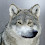### What is the difference between Float and Numeric/Decimal in SQL Server - SQL Server / T-SQL Tutorial Part 33

Float and Real are approximate data types.  Float and Real data types do not store exact values for many numbers.The value can be extremely closed.

Numeric/Decimal are fixed precision data types. It will stored the values with exact precision and scale what you have defined.

If you need to store data where small difference does not matter, you can use Float or Real. But if you need to have exact data such as for financial application. You should be using Numeric/Decimal or Money data type to save exact data, as small difference can impact in calculations.

## Example:

Let's declare two variables and save same data and see what happen to output due to data type of them.

```DECLARE @DecimalVariable DECIMAL(8, 2)

SET @DecimalVariable = 213429.95

DECLARE @FloatVariable FLOAT(24)

SET @FloatVariable = 213429.95

SELECT @DecimalVariable AS DecimalVariable
,@FloatVariable AS FloatVariable```

Difference between Float and Decimal/Numeric in SQL Server - T SQL Tutorial

As we can see in above snapshot, Decimal stored and showed exact values and where we have saved values in float, it rounded the values.

As float/ real data types are approximate data types, Avoid using them using in Where clause specially with = or < > operators.

1.2.